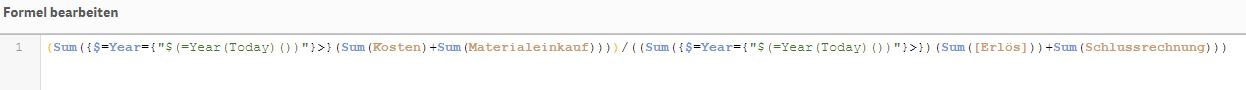# New to Qlik Sense

If you’re new to Qlik Sense, start with this Discussion Board and get up-to-speed quickly.

Announcements
cancel
Showing results for
Did you mean:Creator

## Express KPI of Year (Today)

Dear all,

I want to express the sum of external services per revenue of the current year. The external services is defined by (Kosten + Materialeinkauf), the revenue is defined by (Erlös + Schlussrechnung).

I use the following formula:But the result stays -, so there must be a mistake. Can anyone spot what I did wrong?

Thank you.

Regards

Tom Müller

1 Solution

Accepted SolutionsMaster II

ahh .... got it.. my mistake . didn't see today() had one extra bracket

=(Sum({1<Year={"\$(=Year(Today()))"}>}Kosten)+Sum({1<Year={"\$(=Year(Today()))"}>}Materialeinkauf))

/(Sum({1<Year={"\$(=Year(Today()))"}>}[Erlös])+Sum({1<Year={"\$(=Year(Today()))"}>}Schlussrechnung))

Learning never stops.
13 RepliesMaster II

try removing the '=' after \$

why is '=' there btw? and you can't do sum(sum()) and ' <' is missing . copy paste your expression with a little explanation so that we can help you better

Learning never stops.Creator
Author

(Sum({\$Year={"\$(=Year(Today)())"}>}(Sum(Kosten)+Sum(Materialeinkauf))))/((Sum({\$Year={"\$(=Year(Today)())"}>})(Sum([Erlös]))+Sum(Schlussrechnung)))

But the result is the same, there is no output.

I copied the formula from a youtube QLIK Course, as it worked I expected it to work with my data too.Master II

not sure about the requirement but this is the best i can think off

=(Sum({<Year={"\$(=Year(Today)())"}>}Kosten)+Sum({<Year={"\$(=Year(Today)())"}>}Materialeinkauf))

/(Sum({<Year={"\$(=Year(Today)())"}>}[Erlös])+Sum({<Year={"\$(=Year(Today)())"}>}Schlussrechnung))

Learning never stops.Creator
Author

Now the result is equal to (((Sum(Kosten)+Sum(Materialeinkauf))/((Sum(([Erlös]))+Sum(Schlussrechnung))))), so it includes the set filter.Master II

as i told you i am not aware of your requirement so you have to explain . and you are probably getting data for 2018. check if you have any different years.

Learning never stops.Creator
Author

My requirement is to get a KPI that displays the amount of costs, that external services caused in ratio to my revenue for the current year. So the value of the KPI shall stay the same when I change my filters.

Thanks for your help anyway and sorry for my bad explanations, as english is not my native language the technical terms aren't always in my mind.Master II

Try this

=(Sum({1<Year={"\$(=Year(Today)())"}>}Kosten)+Sum({1<Year={"\$(=Year(Today)())"}>}Materialeinkauf))

/(Sum({1<Year={"\$(=Year(Today)())"}>}[Erlös])+Sum({1<Year={"\$(=Year(Today)())"}>}Schlussrechnung))

It's fine my friend. Knowledge in English is not the only parameter to measure someone capability. Cheers.Learning never stops.Creator
Author

Thanks for the reply. I think we're getting closer. Now the value stays the same, but it shows the result of all given data, but not of the current year. Seems to be logic for me as "1" includes (as far as my knowledge goes) all given data. But I wonder, why "\$" doesn't work, maybe  do I have to change something else in my data editor?Master II

ahh .... got it.. my mistake . didn't see today() had one extra bracket

=(Sum({1<Year={"\$(=Year(Today()))"}>}Kosten)+Sum({1<Year={"\$(=Year(Today()))"}>}Materialeinkauf))

/(Sum({1<Year={"\$(=Year(Today()))"}>}[Erlös])+Sum({1<Year={"\$(=Year(Today()))"}>}Schlussrechnung))

Learning never stops.